# Electronics Toolbox

android-design.nl Download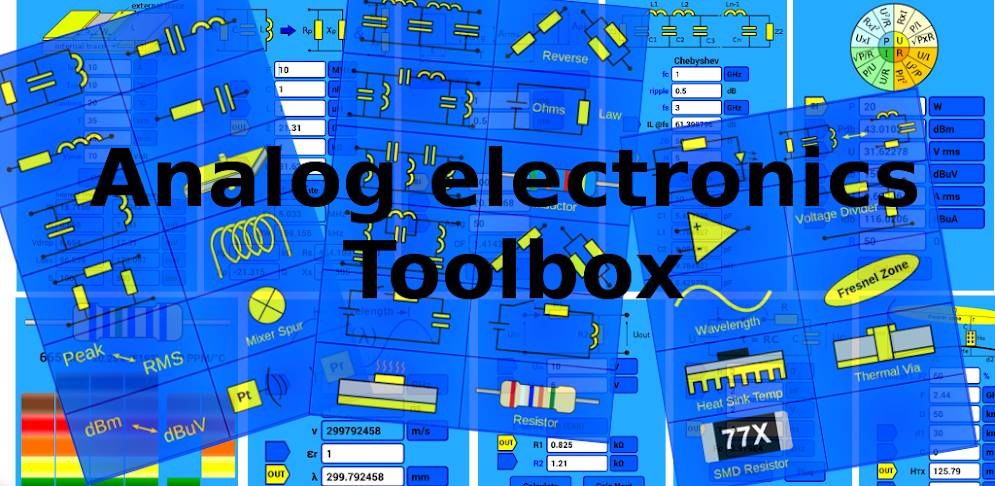## Description

Analog electronics toolbox for electronic engineers, hobbyists and students.

All the tools in this app are also included in the RF & Microwave Toolbox app:
http://play.google.com/store/apps/details?id=mwave.mwcalculator_pro

LIKE and Share us:

Google+: http://gplus.to/androiddesignnl

Feature list:

1) PI, T and L attenuator
2) Power and voltage converter
3) Parallel LCR impedance/resonance
4) Series LCR impedance/resonance
5) Inductor impedance
6) Capacitance impedance
7) Ohm's Law
8) Radio equation calculator (1-way path loss)
9) Air Core Inductor Inductance Calculator
10) Capacitor impedance
11) Noise floor
12) Amplifier cascade (NF, Gain, P1db, OIP2, OIP3)
13) Low Pass filter
14) High Pass filter
15) Helical antenna
16) Band Pass filter
17) Band Stop filter
18) Peak to RMS (peak, RMS, average, CF)
19) Mixer harmonics
20) Resistor Color Code calculator.
21) PCB Trace Width and Clearance Calculator
22) Series and Parallel component (R, L and C) calculations.
23) Reverse Series and Parallel resistor calculations.
24) Inductor Color Code Calculator.
25) Capacitor Charge Calculator.
26) Led Resistor Calculator.
27) Voltage Divider Calculator.
28) Opamp Calculator
29) Wavelength calculator
30) LCR Parallel - Series Conversion Calculator
31) Inductor Charge Calculator.
32) Heat Sink temperature  calculator
33) Thermal via calculator
34) Resistor SMD Code calculator.
35) Bridged T Attenuator Calculator.
36) CR/LR/LCR Calculator (lowpass, highpass, bandpass and bandstop)

Also checkout the RF & Microwave Toolbox which has all the tools of this Electronic Toolbox and the RF & Microwave tools.

Please let me know if there are electronic related tools that you would like to be added.

## Screenshots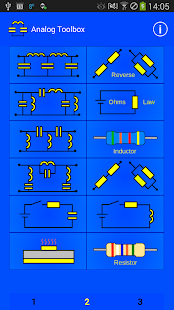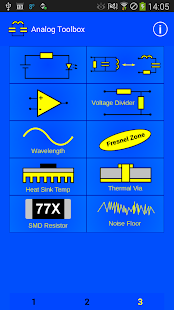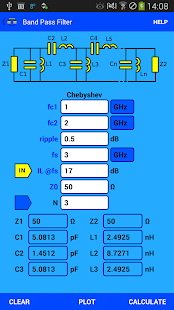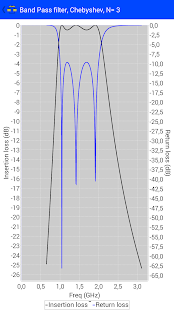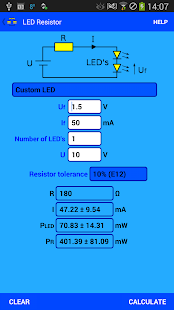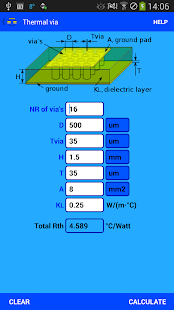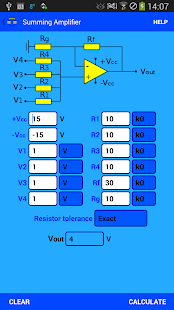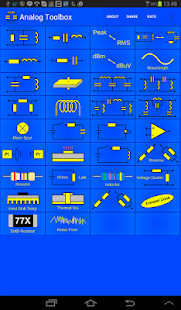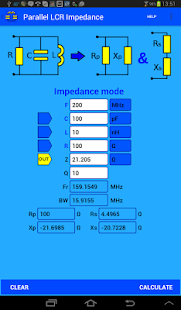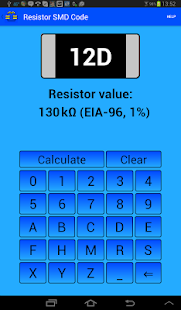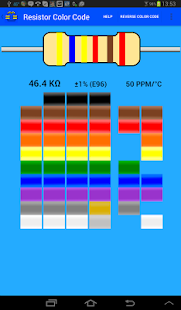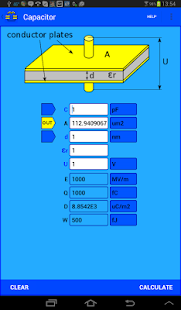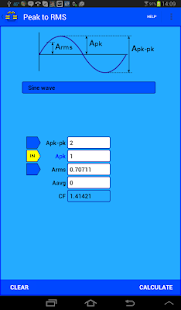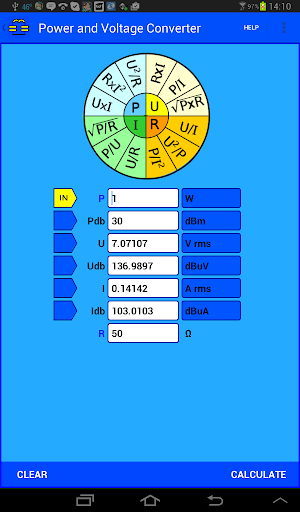## Downloads

Download cracked APK of Electronics Toolbox ( mwave.electronic_toolbox )

 Version 2.51 Uploader Hoster Heisenberg userscloud.comDownload

## Comments

No Comments yet.
You have to login
28366 Apps online